• 2022-02-19 02:11:54

交叉验证在sklearn有相关包，针对多种模型，无需自己写一个构架，用score来寻找好的数据和模型，之后再回测。以下是最简单的实现方法，也很有效：

Scikit-Learn 8 cross validation 交叉验证1 (机器学习 sklearn 教学教程tutorial) - YouTube

Scikit-Learn 9 cross validation 交叉验证2 (机器学习 sklearn 教学教程tutorial) - YouTube

Scikit-Learn 10 cross validation 交叉验证3 (机器学习 sklearn 教学教程tutorial) - YouTubesklearn 机器学习 python
更多相关内容
• 来源：机器学习社区、数据派THU 本文约3400字，建议阅读10分钟 本文与你分享7种最常用的交叉验证技术及其优缺点，提供了每种技术的代码片段。在任何有监督机器学习项目的模型构建阶段，我们...
来源：机器学习社区、数据派THU
本文约3400字，建议阅读10分钟
本文与你分享7种最常用的交叉验证技术及其优缺点，提供了每种技术的代码片段。

在任何有监督机器学习项目的模型构建阶段，我们训练模型的目的是从标记的示例中学习所有权重和偏差的最佳值。

如果我们使用相同的标记示例来测试我们的模型，那么这将是一个方法论错误，因为一个只会重复刚刚看到的样本标签的模型将获得完美的分数，但无法预测任何有用的东西 - 未来的数据，这种情况称为过拟合。

为了克服过度拟合的问题，我们使用交叉验证。所以你必须知道什么是交叉验证？以及如何解决过拟合的问题？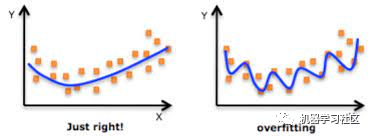#### 什么是交叉验证？

交叉验证是一种用于估计机器学习模型性能的统计方法，它是一种评估统计分析结果如何推广到独立数据集的方法。

#### 它是如何解决过拟合问题的？

在交叉验证中，我们将训练数据生成多个小的训练测试分割，使用这些拆分来调整您的模型。例如，在标准的 k 折交叉验证中，我们将数据划分为 k 个子集。然后，我们在 k-1 个子集上迭代训练算法，同时使用剩余的子集作为测试集。通过这种方式，我们可以在未参与训练的数据上测试我们的模型。

在本文中，我将分享 7 种最常用的交叉验证技术及其优缺点，我还提供了每种技术的代码片段，欢迎收藏学习，喜欢点赞支持。

下面列出了这些技术方法：

• HoldOut 交叉验证

• K-Fold 交叉验证

• 分层 K-Fold交叉验证

• Leave P Out 交叉验证

• 留一交叉验证

• 蒙特卡洛 (Shuffle-Split)

• 时间序列（滚动交叉验证）

#### 1、HoldOut 交叉验证

在这种交叉验证技术中，整个数据集被随机划分为训练集和验证集。根据经验，整个数据集的近 70% 用作训练集，其余 30% 用作验证集。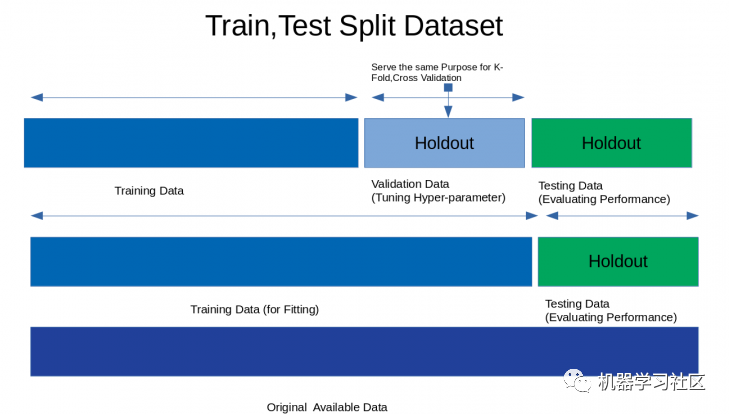优点：

1.快速执行：因为我们必须将数据集拆分为训练集和验证集一次，并且模型将在训练集上仅构建一次，因此可以快速执行。

缺点：

1. 不适合不平衡数据集：假设我们有一个不平衡数据集，它具有“0”类和“1”类。假设 80% 的数据属于“0”类，其余 20% 的数据属于“1”类。在训练集大小为 80%，测试数据大小为数据集的 20% 的情况下进行训练-测试分割。可能会发生“0”类的所有 80% 数据都在训练集中，而“1”类的所有数据都在测试集中。所以我们的模型不能很好地概括我们的测试数据，因为它之前没有看到过“1”类的数据；

2. 大量数据无法训练模型。

在小数据集的情况下，将保留一部分用于测试模型，其中可能具有我们的模型可能会错过的重要特征，因为它没有对该数据进行训练。

代码片段：

from sklearn.datasets import load_iris
from sklearn.model_selection import train_test_split
from sklearn.linear_model import LogisticRegression
from sklearn.metrics import accuracy_score
X=iris.data
Y=iris.target
print("Size of Dataset {}".format(len(X)))
logreg=LogisticRegression()
x_train,x_test,y_train,y_test=train_test_split(X,Y,test_size=0.3,random_state=42)
logreg.fit(x_train,y_train)
predict=logreg.predict(x_test)
print("Accuracy score on training set is {}".format(accuracy_score(logreg.predict(x_train),y_train)))
print("Accuracy score on test set is {}".format(accuracy_score(predict,y_test)))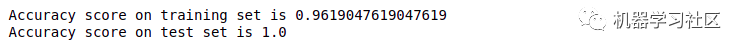#### 2、K 折交叉验证

在这种 K 折交叉验证技术中，整个数据集被划分为 K 个相等大小的部分。每个分区称为一个“折叠”。因此，因为我们有 K 个部分，所以我们称之为 K 折叠。一折用作验证集，其余 K-1 折用作训练集。

该技术重复 K 次，直到每个折叠用作验证集，其余折叠用作训练集。

模型的最终精度是通过取 k-models 验证数据的平均精度来计算的。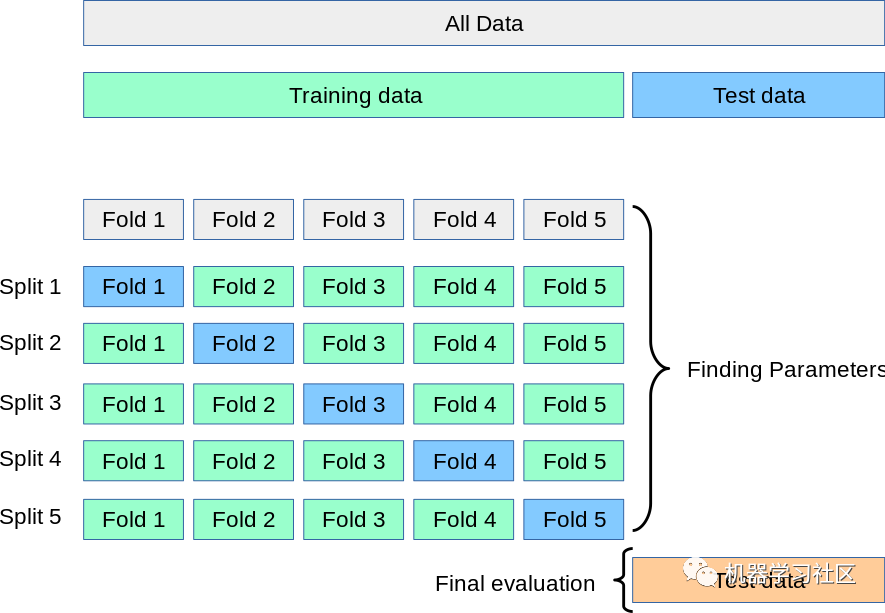优点：

1. 整个数据集既用作训练集又用作验证集。

缺点：

1. 不用于不平衡的数据集：正如在 HoldOut 交叉验证的情况下所讨论的，在 K-Fold 验证的情况下也可能发生训练集的所有样本都没有样本形式类“1”，并且只有 类“0”。验证集将有一个类“1”的样本；

2. 不适合时间序列数据：对于时间序列数据，样本的顺序很重要。但是在 K 折交叉验证中，样本是按随机顺序选择的。

代码片段：

from sklearn.datasets import load_iris
from sklearn.model_selection import cross_val_score,KFold
from sklearn.linear_model import LogisticRegression
X=iris.data
Y=iris.target
logreg=LogisticRegression()
kf=KFold(n_splits=5)
score=cross_val_score(logreg,X,Y,cv=kf)
print("Cross Validation Scores are {}".format(score))
print("Average Cross Validation score :{}".format(score.mean()))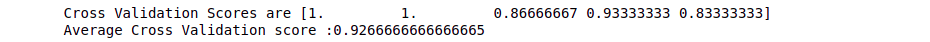#### 3、分层 K 折交叉验证

分层 K-Fold 是 K-Fold 交叉验证的增强版本，主要用于不平衡的数据集。就像 K-fold 一样，整个数据集被分成大小相等的 K-fold。

但是在这种技术中，每个折叠将具有与整个数据集中相同的目标变量实例比率。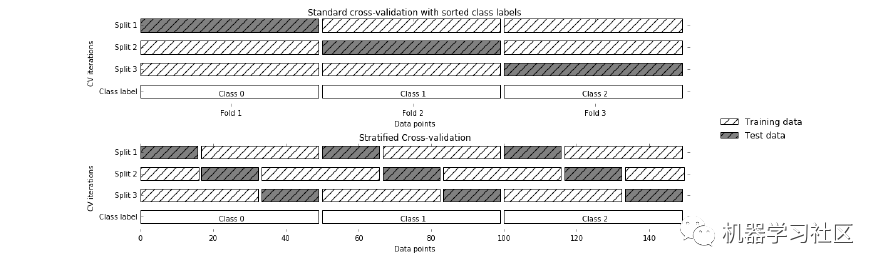优点：

1. 对于不平衡数据非常有效：分层交叉验证中的每个折叠都会以与整个数据集中相同的比率表示所有类别的数据。

缺点：

1. 不适合时间序列数据：对于时间序列数据，样本的顺序很重要。但在分层交叉验证中，样本是按随机顺序选择的。

代码片段：

from sklearn.datasets import load_iris
from sklearn.model_selection import cross_val_score,StratifiedKFold
from sklearn.linear_model import LogisticRegression
X=iris.data
Y=iris.target
logreg=LogisticRegression()
stratifiedkf=StratifiedKFold(n_splits=5)
score=cross_val_score(logreg,X,Y,cv=stratifiedkf)
print("Cross Validation Scores are {}".format(score))
print("Average Cross Validation score :{}".format(score.mean()))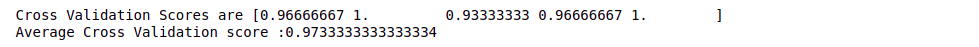#### 4、Leave P Out  交叉验证

Leave P Out 交叉验证是一种详尽的交叉验证技术，其中 p 样本用作验证集，剩余的 n-p 样本用作训练集。

假设我们在数据集中有 100 个样本。如果我们使用 p=10，那么在每次迭代中，10 个值将用作验证集，其余 90 个样本将用作训练集。

重复这个过程，直到整个数据集在 p 样本和 n-p 训练样本的验证集上被划分。

优点：

1. 所有数据样本都用作训练和验证样本。

缺点：

1. 计算时间长：由于上述技术会不断重复，直到所有样本都用作验证集，因此计算时间会更长；

2. 不适合不平衡数据集：与 K 折交叉验证相同，如果在训练集中我们只有 1 个类的样本，那么我们的模型将无法推广到验证集。

代码片段:

from sklearn.model_selection import LeavePOut,cross_val_score
from sklearn.ensemble import RandomForestClassifier
X=iris.data
Y=iris.target
lpo=LeavePOut(p=2)
lpo.get_n_splits(X)
tree=RandomForestClassifier(n_estimators=10,max_depth=5,n_jobs=-1)
score=cross_val_score(tree,X,Y,cv=lpo)
print("Cross Validation Scores are {}".format(score))
print("Average Cross Validation score :{}".format(score.mean()))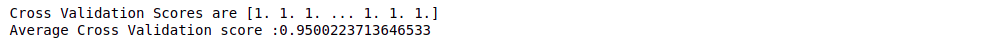#### 5、留一交叉验证

留一交叉验证是一种详尽的交叉验证技术，其中 1 个样本点用作验证集，其余 n-1 个样本用作训练集。

假设我们在数据集中有 100 个样本。然后在每次迭代中，1 个值将用作验证集，其余 99 个样本作为训练集。因此，重复该过程，直到数据集的每个样本都用作验证点。

它与使用 p=1 的 LeavePOut 交叉验证相同。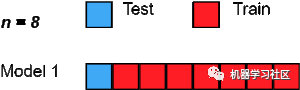代码片段：

from sklearn.datasets import load_iris
from sklearn.ensemble import RandomForestClassifier
from sklearn.model_selection import LeaveOneOut,cross_val_score
X=iris.data
Y=iris.target
loo=LeaveOneOut()
tree=RandomForestClassifier(n_estimators=10,max_depth=5,n_jobs=-1)
score=cross_val_score(tree,X,Y,cv=loo)
print("Cross Validation Scores are {}".format(score))
print("Average Cross Validation score :{}".format(score.mean()))

#### 6、蒙特卡罗交叉验证（Shuffle Split）

蒙特卡罗交叉验证，也称为Shuffle Split交叉验证，是一种非常灵活的交叉验证策略。在这种技术中，数据集被随机划分为训练集和验证集。

我们已经决定了要用作训练集的数据集的百分比和用作验证集的百分比。如果训练集和验证集大小的增加百分比总和不是 100，则剩余的数据集不会用于训练集或验证集。

假设我们有 100 个样本，其中 60% 的样本用作训练集，20% 的样本用作验证集，那么剩下的 20%( 100-(60+20)) 将不被使用。

这种拆分将重复我们必须指定的“n”次。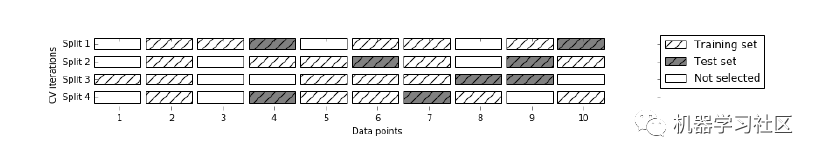优点：

1.我们可以自由使用训练和验证集的大小；

2.我们可以选择重复的次数，而不依赖于重复的折叠次数。

缺点：

1. 可能不会为训练集或验证集选择很少的样本；

2. 不适合不平衡的数据集：在我们定义了训练集和验证集的大小后，所有的样本都是随机选择的，所以训练集可能没有测试中的数据类别 设置，并且该模型将无法概括为看不见的数据。

代码片段：

from sklearn.model_selection import ShuffleSplit,cross_val_score
from sklearn.linear_model import LogisticRegression
logreg=LogisticRegression()
shuffle_split=ShuffleSplit(test_size=0.3,train_size=0.5,n_splits=10)
scores=cross_val_score(logreg,iris.data,iris.target,cv=shuffle_split)
print("cross Validation scores:n {}".format(scores))
print("Average Cross Validation score :{}".format(scores.mean()))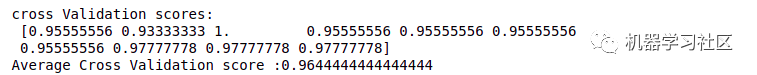#### 7、时间序列交叉验证

什么是时间序列数据？

时间序列数据是在不同时间点收集的数据。由于数据点是在相邻时间段收集的，因此观测值之间可能存在相关性。这是区分时间序列数据与横截面数据的特征之一。

在时间序列数据的情况下如何进行交叉验证？

在时间序列数据的情况下，我们不能选择随机样本并将它们分配给训练集或验证集，因为使用未来数据中的值来预测过去数据的值是没有意义的。

由于数据的顺序对于时间序列相关问题非常重要，所以我们根据时间将数据拆分为训练集和验证集，也称为“前向链”方法或滚动交叉验证。

我们从一小部分数据作为训练集开始。基于该集合，我们预测稍后的数据点，然后检查准确性。

然后将预测样本作为下一个训练数据集的一部分包括在内，并对后续样本进行预测。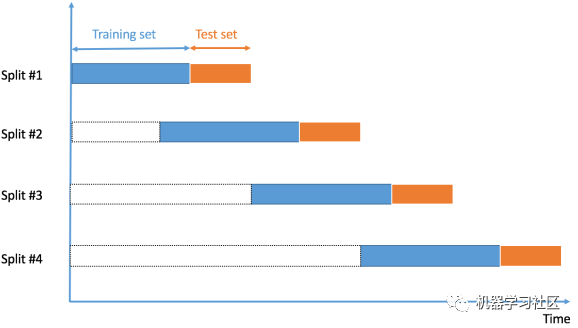优点：

1. 最好的技术之一。

缺点：

1. 不适用于其他数据类型的验证：与其他技术一样，我们选择随机样本作为训练或验证集，但在该技术中数据的顺序非常重要。

代码片段：

import numpy as np
from sklearn.model_selection import TimeSeriesSplit
X = np.array([[1, 2], [3, 4], [1, 2], [3, 4], [1, 2], [3, 4]])
y = np.array([1, 2, 3, 4, 5, 6])
time_series = TimeSeriesSplit()
print(time_series)
for train_index, test_index in time_series.split(X):
print("TRAIN:", train_index, "TEST:", test_index)
X_train, X_test = X[train_index], X[test_index]
y_train, y_test = y[train_index], y[test_index]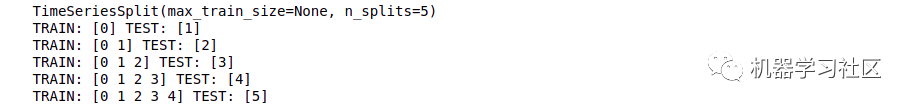### 结论

在本文中，我试图概述各种交叉验证技术的工作原理以及我们在实施这些技术时应牢记的事项，我真诚地希望在这个数据科学之旅中对你有所帮助。

编辑：黄继彦
校对：龚力
推荐阅读：

我的2022届互联网校招分享

我的2021总结

浅谈算法岗和开发岗的区别

互联网校招研发薪资汇总
2022届互联网求职现状，金9银10快变成铜9铁10！！

公众号：AI蜗牛车
保持谦逊、保持自律、保持进步
发送【蜗牛】获取一份《手把手AI项目》（AI蜗牛车著）
发送【1222】获取一份不错的leetcode刷题笔记

发送【AI四大名著】获取四本经典AI电子
展开全文算法 大数据 python 机器学习 人工智能
• 使用 Craven 和 Wahba 的广义交叉验证方法确定最佳平滑参数。 该函数返回平滑样条拟合的输出、平滑参数、估计的方差、估计的 95% 置信区间的大小以及最小化函数的值。 选择平滑参数的通用交叉验证方法应该比 csaps ...matlab
• 针对传统的PHA和FHA方法存在的容易遗漏危险和控制措施，以及人工进行安全性分析的易错、效率低下和难以复用等问题，文章提出一种PHA和FHA结合的安全性交叉验证方法，基于此方法设计和开发了可视化的系统安全性自动...
• 交叉验证是什么？ 在模型建立中，通常有两个数据集：训练集（train）和测试集（test）。训练集用来训练模型；测试集是完全不参与训练的数据，仅仅用来观测测试效果的数据。 一般情况下，训练的结果对于训练集的拟合...

# 交叉验证是什么？

在模型建立中，通常有两个数据集：训练集（train）和测试集（test）。训练集用来训练模型；测试集是完全不参与训练的数据，仅仅用来观测测试效果的数据。

一般情况下，训练的结果对于训练集的拟合程度通常还是挺好的，但是在测试集总的表现却可能不行。比如下面的例子：• 图一的模型是一条线型方程。 可以看到，所有的红点都不在蓝线上，所以导致了错误率很高，这是典型的不拟合的情况
• 图二 的蓝线则更加贴近实际的红点，虽然没有完全重合，但是可以看出模型表示的关系是正确的。
• 图三，所有点都在蓝线上，这时候模型计算出的错误率很低，（甚至将噪音都考虑进去了）。这个模型只在训练集中表现很好，在测试集中的表现就不行。 这是典型的‘过拟合’情况。

所以，训练的模型需要找出数据之间‘真正’的关系，避免‘过拟合’的情况发生。

交叉验证：就是在训练集中选一部分样本用于测试模型。
保留一部分的训练集数据作为验证集/评估集，对训练集生成的参数进行测试，相对客观的判断这些参数对训练集之外的数据的符合程度。

# 留一验证（LOOCV，Leave one out cross validation ）

只从可用的数据集中保留一个数据点，并根据其余数据训练模型。此过程对每个数据点进行迭代，比如有n个数据点，就要重复交叉验证n次。例如下图，一共10个数据，就交叉验证十次优点：

• 适合小样本数据集
• 利用所有的数据点，因此偏差将很低

缺点：

• 重复交叉验证过程n次导致更高的执行时间
• 测试模型有效性的变化大。因为针对一个数据点进行测试，模型的估计值受到数据点的很大影响。如果数据点被证明是一个离群值，它可能导致更大的变化

LOOCC是保留一个数据点，同样的你也可以保留P个数据点作为验证集，这种方法叫LPOCV(Leave P Out Cross Validation)

## LOOCC代码

R

score = list()

LOOCV_function = function(x,label){
for(i in 1:nrow(x)){
training = x[-i,]
model = #... train model on training
validation = x[i,]
pred = predict(model, validation[,setdiff(names(validation),label)])
score[[i]] = rmse(pred, validation[[label]]) # score/error of ith fold
}
return(unlist(score)) # returns a vector
}


# 验证集方法

交叉验证的步骤如下：

1. 保留一个样本数据集， （取出训练集中20%的样本不用）
2. 使用数据集的剩余部分训练模型 （使用另外的80%样本训练模型）
3. 使用验证集的保留样本。（完成模型后，在20%的样本中测试）
4. 如果模型在验证数据上提供了一个肯定的结果，那么继续使用当前的模型。

优点： 简单方便。直接将训练集按比例拆分成训练集和验证集，比如50:50。

缺点： 没有充分利用数据， 结果具有偶然性。如果按50:50分，会损失掉另外50%的数据信息，因为我们没有利用着50%的数据来训练模型。

## 验证集方法代码

Python 使用train_test_split

from sklearn.model_selection import train_test_split
train, validation = train_test_split(data, test_size=0.50, random_state = 5)



train_test_split，划分成train 和test 两部分，其中train用来训练模型，test用来评估模型，模型通过fit方法从train数据集中学习，调用score方法在test集上进行评估。

from sklearn.model_selection import train_test_split
from sklearn.linear_model import LogisticRegression

#已经导入数据
X_train, X_test, y_train, y_test = train_test_split(X, y, test_size=0.2, random_state=20, shuffle=True)

# Logistic Regression
model = LogisticRegression()
model.fit(X_train, y_train)
prediction = model.predict(X_test)
print('The accuracy of the Logistic Regression is: {0}'.format(metrics.accuracy_score(prediction,y_test)))


R

set.seed(101) # Set Seed so that same sample can be reproduced in future also

# Now Selecting 50% of data as sample from total 'n' rows of the data
sample <- sample.int(n = nrow(data), size = floor(.50*nrow(data)), replace = F)
train <- data[sample, ]
test  <- data[-sample, ]


# K折交叉验证（k-fold cross validation）

针对上面通过train_test_split划分，从而进行模型评估方式存在的弊端，提出Cross Validation 交叉验证。

Cross Validation：简言之，就是进行多次train_test_split划分；每次划分时，在不同的数据集上进行训练、测试评估，从而得出一个评价结果；如果是5折交叉验证，意思就是在原始数据集上，进行5次划分，每次划分进行一次训练、评估，最后得到5次划分后的评估结果，一般在这几次评估结果上取平均得到最后的 评分。k-fold cross-validation ，其中，k一般取5或10。1. 训练模型需要在大量的数据集基础上，否则就不能够识别数据中的趋势，导致错误产生
2. 同样需要适量的验证数据点。 验证集太小容易导致误差
3. 多次训练和验证模型。需要改变训练集和验证集的划分，有助于验证模型。
步骤：
4. 随机将整个数据集分成k折；
5. 如图中所示，依次取每一折的数据集作验证集，剩余部分作为训练集
6. 算出每一折测试的错误率
7. 取这里K次的记录平均值 作为最终结果

优点：

• 适合大样本的数据集
• 经过多次划分，大大降低了结果的偶然性，从而提高了模型的准确性。
• 对数据的使用效率更高。train_test_split，默认训练集、测试集比例为3:1。如果是5折交叉验证，训练集比测试集为4:1；10折交叉验证训练集比测试集为9:1。数据量越大，模型准确率越高。

缺点：

• 对数据随机均等划分，不适合包含不同类别的数据集。比如：数据集有5类数据（ABCDE各占20%），抽取出来的也正好是按照类别划分的5类，第一折全是A，第二折全是B……这样就会导致，模型学习到测试集中数据的特点，用BCDE训练的模型去测试A类数据、ACDE的模型测试B类数据，这样准确率就会很低。

如何确定K值？

• 一般情况下3、5是默认选项，常建议用K=10。
• k值越低，就越有偏差；K值越高偏差就越小，但是会受到很大的变化。
• k值越小，就越类似于验证集方法；而k值越大，则越接近LOOCV方法。

## k-fold代码

Python 使用cross_val_score或者KFold

cross_val_score直接将整个交叉验证过程连接起来。

from sklearn.model_selection import cross_val_score
model  = LogisticRegression()
scores = cross_val_score(model,X, y,cv=3) #cv：默认是3折交叉验证，可以修改cv=5，变成5折交叉验证。
print("Cross validation scores:{}".format(scores))
print("Mean cross validation score:{:2f}".format(scores.mean()))


KFold 可以显示具体的划分情况。

from sklearn.model_selection import KFold

kf = KFold(n_splits=5, random_state=None) # 5折

#显示具体划分情况
for train_index, test_index in kf.split(X):
print("Train:", train_index, "Validation:",test_index)
X_train, X_test = X[train_index], X[test_index]
y_train, y_test = y[train_index], y[test_index]

i = 1
for train_index, test_index in kf.split(X, y):
print('\n{} of kfold {}'.format(i,kf.n_splits))
X_train, X_test = X[train_index], X[test_index]
y_train, y_test = y[train_index], y[test_index]
model = LogisticRegression(random_state=1)
model.fit(X_train, y_train)
pred_test = model.predict(X_test)
score = metrics.accuracy_score(y_test, pred_test)
print('accuracy_score', score)
i += 1
#pred_test = model.predict(X_test)
pred = model.predict_proba(X_test)[:, 1]


R code

library(caret)
data(iris)

# Define train control for k fold cross validation
train_control <- trainControl(method="cv", number=10)
# Fit Naive Bayes Model
model <- train(Species~., data=iris, trControl=train_control, method="nb")
# Summarise Results
print(model)


# 分层交叉验证 （Stratified k-fold cross validation）

分层是重新将数据排列组合，使得每一折都能比较好地代表整体。

比如下面这个例子：在一个二分类问题上，原始数据一共有两类（F和M），F：M的数据量比例大概是 1:3；划分了5折，每一折中F和M的比例都保持和原数据一致（1:3）。

这样就避免了随机划分可能产生的的情况，像是一折全是F，其他3折都是M。下图是标准交叉验证和分层交叉验证的区别：
标准交叉验证（即K折交叉验证）：直接将数据分成几折；
分层交叉验证：先将数据分类（class1,2,3)，然后在每个类别中划分三折。## 分层验证代码

Python 使用cross_val_scoreStratifiedKFold

from sklearn.model_selection import StratifiedKFold
skf = StratifiedKFold(n_splits=5,shuffle=False,random_state=0)

# X is the feature set and y is the target
for train_index, test_index in skf.split(X,y):
print("Train:", train_index, "Validation:", test_index)
X_train, X_test = X[train_index], X[test_index]
y_train, y_test = y[train_index], y[test_index]

model = LogisticRegression()
scores = cross_val_score(model,X,y,cv=skf)
print("straitified cross validation scores:{}".format(scores))
print("Mean score of straitified cross validation:{:.2f}".format(scores.mean()))


** R code**

library(caret)
# Folds are created on the basis of target variable
folds <- createFolds(factor(data\$target), k = 10, list = FALSE)


# 重复交叉验证（ k-fold cross validation with repetition）

如果训练集不能很好地代表整个样本总体，分层交叉验证就没有意义了。这时候，可以使用重复交叉验证。

## 重复验证代码

Python：RepeatedKFold重复K折交叉验证

kf = RepeatedKFold(n_splits=5, n_repeats=2, random_state=None)  #默认是5折

for train_index, test_index in kf.split(X):
print("Train:", train_index, "Validation:",test_index)
X_train, X_test = X[train_index], X[test_index]
y_train, y_test = y[train_index], y[test_index]

i = 1
for train_index, test_index in kf.split(X, y):
print('\n{} of kfold {}'.format(i,i))
X_train, X_test = X[train_index], X[test_index]
y_train, y_test = y[train_index], y[test_index]
model = LogisticRegression(random_state=1)
model.fit(X_train, y_train)
pred_test = model.predict(X_test)
score = metrics.accuracy_score(y_test, pred_test)
print('accuracy_score', score)
i += 1
#pred_test = model.predict(X_test)
pred = model.predict_proba(X_test)[:, 1]


在处理实际数据集时，经常会出现测试集和训练集截然不同的情况。因此，可能导致交叉验证结果不一致

在这种情况下，可以使用对抗验证法：总体思路是根据特征分布创建一个分类模型，以检查训练集和测试集之间的相似程度。

步骤：

1. 组合训练集和测试集；
2. 分配0/1标签（0-训练、1-测试）；
3. 建立模型，（如果模型AUC在0.7以上，表示分类器表现较好，也间接说明train 和test 差异度较大
4. 评估二进制分类任务来量化两个数据集的分布是否一致；
5. 找出和测试集最相似的数据样本
6. 构成与测试集最相似的验证集；

优点： 使验证策略在训练集和测试集高度不同的情况下更加可靠。

缺点： 一旦测试集的分布发生变化，验证集可能不再适合评估模型。

## 对抗验证代码

#1. 将目标变量删除
train.drop(['target'], axis = 1, inplace = True)

#2. 创建新的目标变量：训练集为1；测试集为0
train['is_train'] = 1
test['is_train'] = 0

#3. 合并训练集和测试集
df = pd.concat([train, test], axis = 0)

#4. 使用新变量训练分类模型，并预测概率
y = df['is_train']; df.drop('is_train', axis = 1, inplace = True)
# Xgboost parameters
xgb_params = {'learning_rate': 0.05,
'max_depth': 4,
'subsample': 0.9,
'colsample_bytree': 0.9,
'objective': 'binary:logistic',
'silent': 1,
'n_estimators':100,
'gamma':1,
'min_child_weight':4}
clf = xgb.XGBClassifier(**xgb_params, seed = 10)
probs = clf.predict_proba(x1)[:,1]

#5. 使用步骤4中计算的概率对序列集进行排序，并将前n%个样本/行作为验证集（n%是您希望保留在验证集中的序列集的分数）
new_df = pd.DataFrame({'id':train.id, 'probs':probs})
new_df = new_df.sort_values(by = 'probs', ascending=False) # 30% validation set
val_set_ids = new_df.iloc[1:np.int(new_df.shape*0.3),1]
#val_set_ids将为提供列训练集的ID，这些ID将构成与测试集最相似的验证集。


# 时间序列的交叉验证(Cross Validation for time series）

对于时间序列的数据集，不能像上述方法一样随机地划分验证集。为了解决时间序列的预测问题，可以尝试时间序列交叉验证：采用正向链接的策略，即按照时间顺序划分每一折的数据集。

假设我们有一个时间序列，表示在n年内消费者对某一产品的年需求量。我们逐步选择新的训练集和测试集。我们从一个最小的训练集开始（这个训练集具有拟合模型所需的最少观测数）逐步地，每次都会更换训练集和测试集。在大多数情况下，不必一个个点向前移动，可以设置一次跨5个点/10个点。在回归问题中，可以使用以下代码执行交叉验证。

## 时间序列代码

pythonTimeSeriesSplit

from sklearn.model_selection import TimeSeriesSplit
X = np.array([[1, 2], [3, 4], [1, 2], [3, 4]])
y = np.array([1, 2, 3, 4])
tscv = TimeSeriesSplit(n_splits=3)

for train_index, test_index in tscv.split(X):
print("Train:", train_index, "Validation:", val_index)
X_train, X_test = X[train_index], X[val_index]
y_train, y_test = y[train_index], y[val_index]

TRAIN:  TEST: 
TRAIN: [0 1] TEST: 
TRAIN: [0 1 2] TEST: 


R code

library(fpp)
library(forecast)
e <- tsCV(ts, Arima(x, order=c(2,0,0), h=1) #CV for arima model
sqrt(mean(e^2, na.rm=TRUE)) # RMSE
#h ＝1意味着我们只接受1步超前预报的误差。
#（h=4）4步前进误差如下图所示。如果想评估多步预测的模型，可以使用此选项。参考链接：https://www.analyticsvidhya.com/blog/2018/05/improve-model-performance-cross-validation-in-python-r/
其他网上找到的有关文章：
关于时间序列的：https://zhuanlan.zhihu.com/p/99674163
关于对抗验证的：https://zhuanlan.zhihu.com/p/137580733

展开全文机器学习 python
• 学习器在测试集上的误差我们通常称作“泛化误差”。要想得到“泛化误差”首先得将数据集划分为训练集和测试集。那么怎么划分呢？...KF=KFold(n_splits=2) #建立4折交叉验证方法 查一下KFold函数的参数
• 机器学习中的交叉验证方法，可以用于分类结果的验证，参数的选择验证等模式识别
• 在任何有监督机器学习项目的模型构建阶段，我们训练模型的目的是从标记的示例中学习所有权重和偏差的最佳值。如果我们使用相同的标记示例来测试我们的模型，那么这将是一个方法论错误，因为一个只会重复...

在任何有监督机器学习项目的模型构建阶段，我们训练模型的目的是从标记的示例中学习所有权重和偏差的最佳值。

如果我们使用相同的标记示例来测试我们的模型，那么这将是一个方法论错误，因为一个只会重复刚刚看到的样本标签的模型将获得完美的分数，但无法预测任何有用的东西 - 未来的数据，这种情况称为过拟合。

为了克服过度拟合的问题，我们使用交叉验证。所以你必须知道什么是交叉验证？以及如何解决过拟合的问题？#### 什么是交叉验证？

交叉验证是一种用于估计机器学习模型性能的统计方法，它是一种评估统计分析结果如何推广到独立数据集的方法。

#### 它是如何解决过拟合问题的？

在交叉验证中，我们将训练数据生成多个小的训练测试分割，使用这些拆分来调整您的模型。例如，在标准的 k 折交叉验证中，我们将数据划分为 k 个子集。然后，我们在 k-1 个子集上迭代训练算法，同时使用剩余的子集作为测试集。通过这种方式，我们可以在未参与训练的数据上测试我们的模型。

在本文中，我将分享 7 种最常用的交叉验证技术及其优缺点，我还提供了每种技术的代码片段，欢迎收藏学习，喜欢点赞支持。

下面列出了这些技术方法：

• HoldOut 交叉验证

• K-Fold 交叉验证

• 分层 K-Fold交叉验证

• Leave P Out 交叉验证

• 留一交叉验证

• 蒙特卡洛 (Shuffle-Split)

• 时间序列（滚动交叉验证）

#### 1、HoldOut 交叉验证

在这种交叉验证技术中，整个数据集被随机划分为训练集和验证集。根据经验，整个数据集的近 70% 用作训练集，其余 30% 用作验证集。优点

1.快速执行：因为我们必须将数据集拆分为训练集和验证集一次，并且模型将在训练集上仅构建一次，因此可以快速执行。

缺点

1. 不适合不平衡数据集：假设我们有一个不平衡数据集，它具有“0”类和“1”类。假设 80% 的数据属于“0”类，其余 20% 的数据属于“1”类。在训练集大小为 80%，测试数据大小为数据集的 20% 的情况下进行训练-测试分割。可能会发生“0”类的所有 80% 数据都在训练集中，而“1”类的所有数据都在测试集中。所以我们的模型不能很好地概括我们的测试数据，因为它之前没有看到过“1”类的数据。

2. 大量数据无法训练模型。

在小数据集的情况下，将保留一部分用于测试模型，其中可能具有我们的模型可能会错过的重要特征，因为它没有对该数据进行训练。

代码片段

from sklearn.datasets import load_iris
from sklearn.model_selection import train_test_split
from sklearn.linear_model import LogisticRegression
from sklearn.metrics import accuracy_score
X=iris.data
Y=iris.target
print("Size of Dataset {}".format(len(X)))
logreg=LogisticRegression()
x_train,x_test,y_train,y_test=train_test_split(X,Y,test_size=0.3,random_state=42)
logreg.fit(x_train,y_train)
predict=logreg.predict(x_test)
print("Accuracy score on training set is {}".format(accuracy_score(logreg.predict(x_train),y_train)))
print("Accuracy score on test set is {}".format(accuracy_score(predict,y_test)))#### 2、K 折交叉验证

在这种 K 折交叉验证技术中，整个数据集被划分为 K 个相等大小的部分。每个分区称为一个“折叠”。因此，因为我们有 K 个部分，所以我们称之为 K 折叠。一折用作验证集，其余 K-1 折用作训练集。

该技术重复 K 次，直到每个折叠用作验证集，其余折叠用作训练集。

模型的最终精度是通过取 k-models 验证数据的平均精度来计算的。优点

1. 整个数据集既用作训练集又用作验证集：

缺点

1. 不用于不平衡的数据集：正如在 HoldOut 交叉验证的情况下所讨论的，在 K-Fold 验证的情况下也可能发生训练集的所有样本都没有样本形式类“1”，并且只有 类“0”。验证集将有一个类“1”的样本。

2. 不适合时间序列数据：对于时间序列数据，样本的顺序很重要。但是在 K 折交叉验证中，样本是按随机顺序选择的。

代码片段

from sklearn.datasets import load_iris
from sklearn.model_selection import cross_val_score,KFold
from sklearn.linear_model import LogisticRegression
X=iris.data
Y=iris.target
logreg=LogisticRegression()
kf=KFold(n_splits=5)
score=cross_val_score(logreg,X,Y,cv=kf)
print("Cross Validation Scores are {}".format(score))
print("Average Cross Validation score :{}".format(score.mean()))#### 3、分层 K 折交叉验证

分层 K-Fold 是 K-Fold 交叉验证的增强版本，主要用于不平衡的数据集。就像 K-fold 一样，整个数据集被分成大小相等的 K-fold。

但是在这种技术中，每个折叠将具有与整个数据集中相同的目标变量实例比率。优点

1. 对于不平衡数据非常有效：分层交叉验证中的每个折叠都会以与整个数据集中相同的比率表示所有类别的数据。

缺点

1. 不适合时间序列数据：对于时间序列数据，样本的顺序很重要。但在分层交叉验证中，样本是按随机顺序选择的。

代码片段

from sklearn.datasets import load_iris
from sklearn.model_selection import cross_val_score,StratifiedKFold
from sklearn.linear_model import LogisticRegression
X=iris.data
Y=iris.target
logreg=LogisticRegression()
stratifiedkf=StratifiedKFold(n_splits=5)
score=cross_val_score(logreg,X,Y,cv=stratifiedkf)
print("Cross Validation Scores are {}".format(score))
print("Average Cross Validation score :{}".format(score.mean()))#### 4、Leave P Out  交叉验证

Leave P Out 交叉验证是一种详尽的交叉验证技术，其中 p 样本用作验证集，剩余的 np 样本用作训练集。

假设我们在数据集中有 100 个样本。如果我们使用 p=10，那么在每次迭代中，10 个值将用作验证集，其余 90 个样本将用作训练集。

重复这个过程，直到整个数据集在 p-样本和 n-p 训练样本的验证集上被划分。

优点

所有数据样本都用作训练和验证样本。

缺点

1. 计算时间长：由于上述技术会不断重复，直到所有样本都用作验证集，因此计算时间会更长。

2. 不适合不平衡数据集：与 K 折交叉验证相同，如果在训练集中我们只有 1 个类的样本，那么我们的模型将无法推广到验证集。

代码片段

from sklearn.model_selection import LeavePOut,cross_val_score
from sklearn.ensemble import RandomForestClassifier
X=iris.data
Y=iris.target
lpo=LeavePOut(p=2)
lpo.get_n_splits(X)
tree=RandomForestClassifier(n_estimators=10,max_depth=5,n_jobs=-1)
score=cross_val_score(tree,X,Y,cv=lpo)
print("Cross Validation Scores are {}".format(score))
print("Average Cross Validation score :{}".format(score.mean()))#### 5、留一交叉验证

留一交叉验证是一种详尽的交叉验证技术，其中 1 个样本点用作验证集，其余 n-1 个样本用作训练集。

假设我们在数据集中有 100 个样本。然后在每次迭代中，1 个值将用作验证集，其余 99 个样本作为训练集。因此，重复该过程，直到数据集的每个样本都用作验证点。

它与使用 p=1 的 LeavePOut 交叉验证相同。「代码片段」

from sklearn.datasets import load_iris
from sklearn.ensemble import RandomForestClassifier
from sklearn.model_selection import LeaveOneOut,cross_val_score
X=iris.data
Y=iris.target
loo=LeaveOneOut()
tree=RandomForestClassifier(n_estimators=10,max_depth=5,n_jobs=-1)
score=cross_val_score(tree,X,Y,cv=loo)
print("Cross Validation Scores are {}".format(score))
print("Average Cross Validation score :{}".format(score.mean()))

#### 6、蒙特卡罗交叉验证（Shuffle Split）

蒙特卡罗交叉验证，也称为Shuffle Split交叉验证，是一种非常灵活的交叉验证策略。在这种技术中，数据集被随机划分为训练集和验证集。

我们已经决定了要用作训练集的数据集的百分比和用作验证集的百分比。如果训练集和验证集大小的增加百分比总和不是 100，则剩余的数据集不会用于训练集或验证集。

假设我们有 100 个样本，其中 60% 的样本用作训练集，20% 的样本用作验证集，那么剩下的 20%( 100-(60+20)) 将不被使用。

这种拆分将重复我们必须指定的“n”次。优点

1.我们可以自由使用训练和验证集的大小。

2.我们可以选择重复的次数，而不依赖于重复的折叠次数。

缺点

1. 可能不会为训练集或验证集选择很少的样本。

2. 不适合不平衡的数据集：在我们定义了训练集和验证集的大小后，所有的样本都是随机选择的，所以训练集可能没有测试中的数据类别 设置，并且该模型将无法概括为看不见的数据。

代码片段

from sklearn.model_selection import ShuffleSplit,cross_val_score
from sklearn.linear_model import LogisticRegression
logreg=LogisticRegression()
shuffle_split=ShuffleSplit(test_size=0.3,train_size=0.5,n_splits=10)
scores=cross_val_score(logreg,iris.data,iris.target,cv=shuffle_split)
print("cross Validation scores:n {}".format(scores))
print("Average Cross Validation score :{}".format(scores.mean()))#### 7、时间序列交叉验证

什么是时间序列数据？

时间序列数据是在不同时间点收集的数据。由于数据点是在相邻时间段收集的，因此观测值之间可能存在相关性。这是区分时间序列数据与横截面数据的特征之一。

在时间序列数据的情况下如何进行交叉验证？

在时间序列数据的情况下，我们不能选择随机样本并将它们分配给训练集或验证集，因为使用未来数据中的值来预测过去数据的值是没有意义的。

由于数据的顺序对于时间序列相关问题非常重要，所以我们根据时间将数据拆分为训练集和验证集，也称为“前向链”方法或滚动交叉验证。

我们从一小部分数据作为训练集开始。基于该集合，我们预测稍后的数据点，然后检查准确性。

然后将预测样本作为下一个训练数据集的一部分包括在内，并对后续样本进行预测。优点

最好的技术之一。

缺点

不适用于其他数据类型的验证：与其他技术一样，我们选择随机样本作为训练或验证集，但在该技术中数据的顺序非常重要。

代码片段

import numpy as np
from sklearn.model_selection import TimeSeriesSplit
X = np.array([[1, 2], [3, 4], [1, 2], [3, 4], [1, 2], [3, 4]])
y = np.array([1, 2, 3, 4, 5, 6])
time_series = TimeSeriesSplit()
print(time_series)
for train_index, test_index in time_series.split(X):
print("TRAIN:", train_index, "TEST:", test_index)
X_train, X_test = X[train_index], X[test_index]
y_train, y_test = y[train_index], y[test_index]### 结论

在本文中，我试图概述各种交叉验证技术的工作原理以及我们在实施这些技术时应牢记的事项，我真诚地希望在这个数据科学之旅中对你有所帮助。

往期精彩回顾

适合初学者入门人工智能的路线及资料下载机器学习及深度学习笔记等资料打印机器学习在线手册深度学习笔记专辑《统计学习方法》的代码复现专辑
AI基础下载黄海广老师《机器学习课程》视频课黄海广老师《机器学习课程》711页完整版课件

本站qq群955171419，加入微信群请扫码：展开全文人工智能 大数据 算法 python 机器学习
• 提供交叉验证神经网络matlab代码，供大家学习。
• 引用自http://blog.sina.com.cn/s/blog_67331d610102vl2t.html中内容交叉验证(CrossValidation)方法思想 交叉验证(Cross Validation)简称为CV，是用来验证分类器的性能一种统计分析方法,基本思想是把在某种意义下将...
• 电信设备-多重无线通信协议的交叉验证方法及其装置.zip
• 2、通过交叉验证方法，逐个来验证。 很显然我是属于后者所以我需要在这里记录一下 sklearn 的 cross_val_score： 我使用是cross_val_score方法，在sklearn中可以使用这个方法交叉验证的原理不好表述下面随手画了...
• 监督学习算法预测性能比较的正则化交叉验证方法之软件工程研究.docxC语言
• 1.常用的判断方法是从训练集中随机选一部分作为一个验证集，采用K折交叉验证的方式，用训练集训练的同时在验证集上测试算法效果。在缺少有效预防欠拟合和过拟合措施的情况下，随着模型拟合能力的增强，错误率在训练...
• 基本流程： 1、计算测试实例到所有训练集实例的距离；...交叉验证： 对每一个k，使用验证集计算，记录k对应的错误次数，取错误数最小的k # -*- coding: utf-8 -*- import os import pandas as pd import ma...iris数据集
• 来源：机器学习社区、数据派THU 本文约3400字，建议阅读10分钟 本文与你分享7种最常用的交叉验证技术及其优缺点，提供了每种技术的代码片段。在任何有监督机器学习项目的模型构建阶段，我们...大数据 算法 python 机器学习 人工智能
• 【机器学习】交叉验证详细解释+10种常见的验证方法具体代码实现+可视化图 一、使用背景 由于在训练集上，通过调整参数设置使估计器的性能达到了最佳状态；但在测试集上可能会出现过拟合的情况。 此时，测试集上的...机器学习 ROC曲线
• 下面列出了这些技术方法： HoldOut 交叉验证 K-Fold 交叉验证 分层 K-Fold交叉验证 Leave P Out 交叉验证 留一交叉验证 蒙特卡洛 (Shuffle-Split) 时间序列（滚动交叉验证） 1、HoldOut 交叉验证 在这种交叉验证...算法 python 机器学习 人工智能 深度学习
• 交叉验证是进行模型比较的一种有效方法。 它的基本原理如下(Kohavi，1995）： （1）把原始数据集分解成 r个大小近似相等的子数据集。 （2）把第一个子数据集作为验证数据集，把其余r-1个子数据集合并后用于估计...r语言 数据 模型选择
• 什么是交叉验证法？ 为什么用交叉验证法？ 主要有哪些方法？优缺点？ 各方法应用举例？ 什么是交叉验证法？ 它的基本思想就是将原始数据（dataset）进行分组，一部分做为训练集来训练模型，另一部分做为测试集来...
• 来源：机器学习社区 本文约3400字，建议阅读10分钟 本文与你分享7种最常用的交叉验证技术及其优缺点，提供了每种技术的代码片段。在任何有监督机器学习项目的模型构建阶段，我们训练模型的目的...大数据 算法 python 机器学习 人工智能
• klearn交叉验证函数 ‘BaseCrossValidator’, ’GridSearchCV’, ‘TimeSeriesSplit’, ‘KFold’, ‘GroupKFold’, ‘GroupShuffleSplit’, ‘LeaveOneGroupOut’, ‘LeaveOneOut’, ‘LeavePGroupsOut’, ...
• 交叉验证原理与常用方法 scikitlearn交叉验证评估 交叉验证：评估估算器的表现 在交叉验证中数据集一般可以分为训练集和测试集，其中训练集的某一折用于作为验证集，这样有利于充分利用数据，但是同样提升了计算量。...
• 选自toward data science作者：Courtney Cochrane编译：机器之心本文简要讲解了交叉验证和嵌套交叉验证，并介绍了针对单个时序数据和多个时序数...
• 交叉验证(CrossValidation)方法思想 以下简称交叉验证(Cross Validation)为CV.CV是用来验证分类器的性能一种统计分析方法,基本思想是把在某种意义下将原始数据(dataset)进行分组,一部分做为训练集(train set),另一...
• 1.我要做交叉验证，需要每个训练集和测试集都保持相同的样本分布比例，直接用sklearn提供的KFold并不能满足这个需求。 2.将生成的交叉验证数据集保存成CSV文件，而不是直接用sklearn训练分类模型。 3.在编码过程中有...
• 在任何有监督机器学习项目的模型构建阶段，我们训练模型的目的是从标记的示例中学习所有权重和偏差的最佳值。...交叉验证是一种用于估计机器学习模型性能的统计方法，它是一种评估统计分析结果如何推广到独立数据python 机器学习
• 1、交叉验证 通俗的讲就是将样本均分为几等份，拿出某一（几）份作为测试样本，剩下的作为训练样本，去预测得到相应指标（准确率，召回率，f1等），再抽取另一份（几份）作为测试样本，剩下的作为训练样本，去预测......